# SCOUTer demo

library(SCOUTer)
#> Loading required package: ggplot2
#> Loading required package: ggpubr

# Exploring the reference dataset

## Using PCA models

The demo matrix X (already loaded with the package) will be used for all the examples. First we build the PCA model (PCA - Model Building, PCA-MB).

X <- as.matrix(X)
pcamodel_ref <- pcamb_classic(X, 2, 0.05, "cent")

Once a PCA model is obtained, data sets can be projected onto it. This is the PCA - Model Exploitation ( PCA-ME ) framework. The function pcame.R returns a list with the results of this projection.

pcax <- pcame(X, pcamodel_ref)

## Distance plot and score plot

Functions distplot.R and scoreplot.R are used to obtain the distance plot and the score plot respectively. However, dscplot.R can be used to obtain both as subplots of the same figure.

dscplot(X, pcamodel_ref)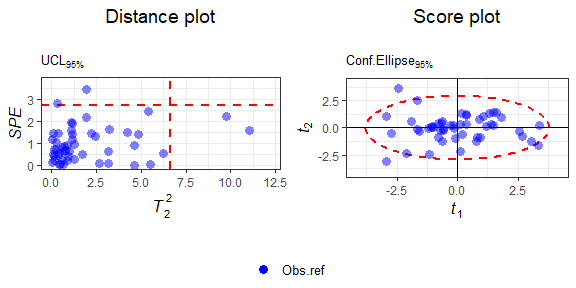Distance plot (left) and score plot (right) of the reference data and PCA model.

This is the default layout. If a vertical disposition is preferred, then:

dscplot(X, pcamodel_ref, nrow = 2, ncol = 1)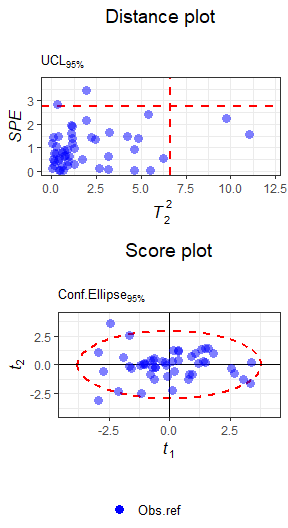Distance plot (left) and score plot (right) of the reference data and PCA model with vertical layout.

Alternatively, if, for instance, only the distance plot is required:

distplot(X, pcamodel_ref)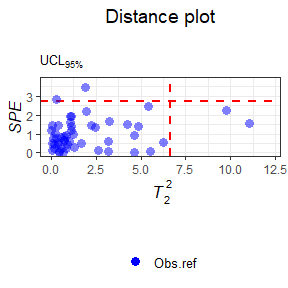Distance plot of the reference data and PCA model.

Note that the dataset and the PCA model are used as inputs, instead of the projection results. This is because all these functions innerly perform the PCA-ME step.

## Other plots

The SCOUTer package includes other graphical functions. The function obscontribpanel.R, ensembles all of them in one figure. Given an observation of interest, it displays information about the SPE, the T2 and the contributions to them.

In this example, the information about the observation with the maximal SPE will be displayed.

obscontribpanel(pcax, pcamodel_ref, which.max(pcax$SPE))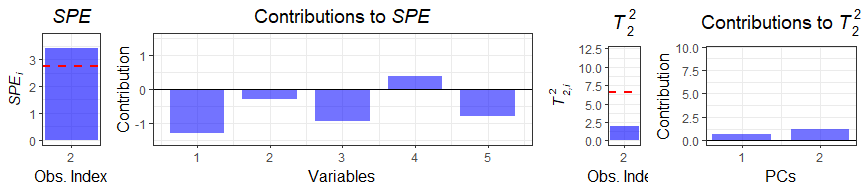Contribution panel for SPE and T2 values. This layout can be divided in two sections: information about the SPE and information about the T2. These parts can be individually obtained as plots by functions speinfo.R and ht2info.R. Alternatively, another way of dividing the elemtns of the figure is the bar plot types. On one hand, there are bar plots with the reference of the Upper Control Limit. These are obtained by the barwithucl.R function. On the other hand, contribution plots are obtained with the custombar.R function. Both have customizable label options. # Display SPE of the first observation barwithucl(pcax$SPE, 1, pcamodel_ref$limspe, plotname = "SPE")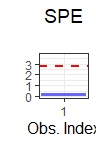Bar plot with the SPE value of an observation (bar) and the UCL according to the PCA model (red line) # Display contributions to the SPE of the same observation custombar(pcax$E, 1, plotname = "Contributions to SPE")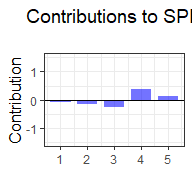Bar plot with the contributions of each variable (error vector, e) to the SPE value

# Simulating outliers

Simulation can be performed using the scout.R function. The following examples will illustrate the three main types of SCOUTer simulation modes: simple, steps and grid.

## Simple mode

An observation is chosen randomly from X and the scout.R function is used in order to shift it obtaining a new observation with target values equal to 40 for both statistics.

set.seed(1218) # ensure always the same result
indsel <- sample(1:nrow(X), 1)
x <- t(as.matrix(X[indsel,]))
x.out <- scout(x, pcamodel_ref, T2.y = 40, SPE.y = 40, mode = "simple")

In order to shift a set of observations, the target values must be vectors with the target value corresponding to each observation in the input data matrix.

Now, all observations from X will be shifted, generating another data set with T2 = 40 for all observations.

n <- nrow(X)
X.T2.40 <- scout(X, pcamodel_ref, T2.y = matrix(40, n, 1), mode = "simple")

In order to display both datasets together, the argument obstag in the dscplot.R function can be used.

X.all <- rbind(X, X.T2.40$X) tag.all <- dotag(X, X.T2.40$X)
dscplot(X.all, pcamodel_ref, obstag = tag.all)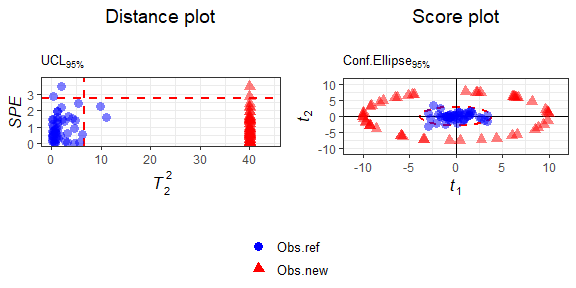Distance plot (left) and score plot (right) of the data simulated with simple mode.

## Steps mode

In this case it is included an intermediate step between the initial values and the target ones, which is the incremental variation of the SPE and the T2. There are two new parameters to set:

• The number of steps to perform until reaching the target values for each statistic.

• The spacing between steps (gamma), which tunes the linearity of the spacing. If any value is provided, a linear spacing (gt2 and gspe input arguments keep their default value) is performed.

x.out.steps <- scout(x, pcamodel_ref, T2.y = 40, SPE.y = 40, nsteps = 10, mode = "steps")
x.all <- rbind(x, x.out.steps$X) tag.all <- dotag(x, x.out.steps$X)
dscplot(x.all, pcamodel_ref, obstag = tag.all)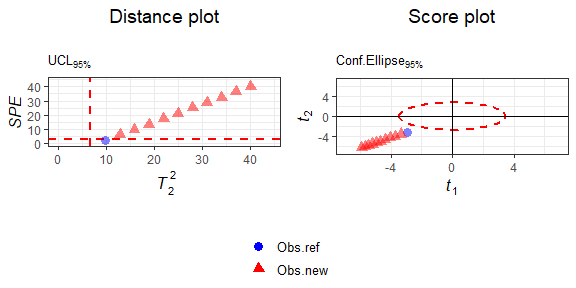Distance plot (left) and score plot (right) of the data simulated with steps mode.

## Grid mode

Finally, in this case, instead of increasing in a step-wise joint manner both the SPE and the T2, a grid of steps is created. This implies simulating all combinations of { SPE, T2 } along their steps. Thus, nsteps.spe x nsteps.t2 sets are created.

In this last case, a grid with 3 steps for the T2 and 2 steps for the SPE is simulated. Moreover, steps will be non-linearly spaced, by setting the input arguments gspe and gt2 to values different to 1.

x.out.grid <- scout(x, pcamodel_ref, T2.y = 40, SPE.y = 40, nsteps.spe = 2, nsteps.t2 = 3, gspe = 3, gt2 =0.3, mode = "grid")
x.all <- rbind(x, x.out.grid$X) tag.all <- dotag(x, x.out.grid$X)
dscplot(x.all, pcamodel_ref, obstag = tag.all)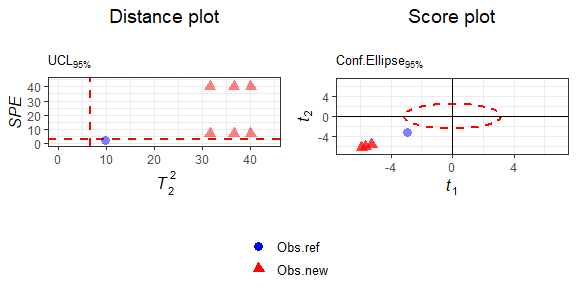Distance plot (left) and score plot (right) of the data simulated with grid mode.